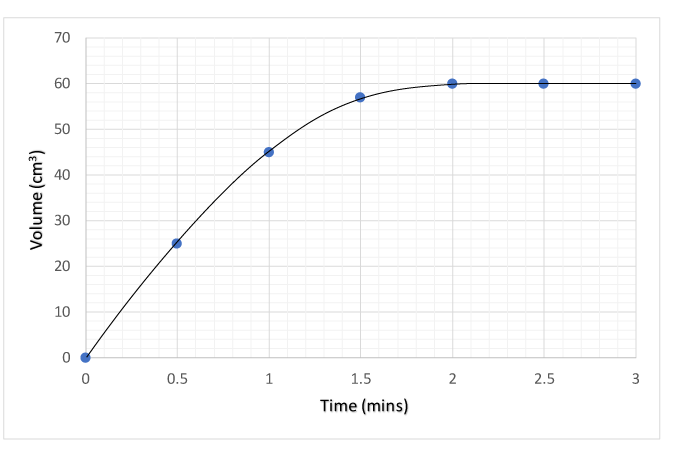Chemistry µGCSE:

Rates of Reaction

10 quick questions - for GCSE and iGCSE

 10 minutes maximum! (can you do it in 5?) 1. The rate of a chemical reaction ? increases decreases stays the same as the reaction proceeds. 2. Which of the following factors would increase the rate of a reaction? (I) powdering a solid (II) increasing the temperature of a solution (III) decreasing the concentration of solution A. (I) and (II) B. (I) and (III) C. (II) and (III) D. (I), (II) and (III) ? A B C D 3. Increasing the pressure of a mixture of hydrogen and oxygen gases would …. A. ….decrease the rate of reaction B. …..increase the rate of reaction C. …..not affect the rate of reaction D. …..increase the volume of the mixture ? A B C D Q4-6. A student investigated the effect of increasing the temperature on the rate of reaction between magnesium and hydrochloric acid. The equation for this reaction is: Mg(s) + 2HCl(aq) → MgCl2(aq) + H2(g) 4. To ensure a fair test the student should use the same …. A.  temperature, mass of magnesium and concentration of acid B.  temperature, mass of magnesium and volume of acid C.  mass of magnesium, concentration and volume of acid D.  mass of magnesium, volume of acid and temperature ? A B C D 5. The initial rate of reaction increased with temperature. The initial rate could be decreased by .. A. …using powdered magnesium B. …decreasing acid volume                               C. …increasing acid volume D. …decreasing acid concentration ? A B C D 6. The rate of this reaction could be determined by recording the… A. ….volume of gas at regular time intervals B. ….time for the magnesium to disappear C. ….time to collect a fixed volume of gas D. ….all of the above ? A B C D Q7-8. In the reaction between marble chips and hydrochloric acid, one way to increase the rate of reaction is to increase the acid concentration. CaCO3(s) + 2HCl(aq) → CaCl2(aq) + H2O(l) + CO2(g) 7. Rate can also be increased by ….. A. increasing temperature B. crushing the marble chips C. adding a suitable catalyst D. all of the above ? A B C D 8. The best reason for the reaction rate increasing with concentration is…… A. …more reactant particles which increases the frequency of collisions B. …more reactant particles which increases the energy of the collisions C. …more reactant particles per unit volume which increases the frequency of collisions D. …more reactant particles per unit volume which increases the energy of the collisions ? A B C D Q9-10. The following graph was obtained by reacting excess magnesium powder with 50cm3 0.1mol/dm3 hydrochloric acid at 250C. The volume of gas produced was measured over a 3 minute period:9. A steeper graph which produces the same final volume of gas would be obtained by … A. ….using the same mass of magnesium ribbon instead of powder B. ….using the same volume of a more concentrated acid C. ….increasing the temperature D. ….decreasing the temperature ? A B C D 10. Repeating the experiment with 100cm3 of 0.1mol/dm3 hydrochloric acid at 250C would produce a graph which …… A. …. is steeper and finishes at the same final volume B. …. is steeper but produces a greater final volume C. ….has the same gradient and finishes at the same final volume D. ….has the same gradient but produces a greater final volume ? A B C D